# Secure Hash Algorithm-2 formed on DNA

## Abstract

We present a new version of the Secure Hash Algorithm-2 (SHA-2) formed on artificial sequences of deoxyribonucleic acid (DNA). This article is the first attempt to present the implementation of SHA-2 using DNA data processing. We called the new version DNSHA-2. We present new operations on an artificial DNA sequence, such as (1) $$\bar {R}^{k}(\alpha)$$ and $$\bar {L}^{k}(\alpha)$$ to mimic the right and left shift by k bits, respectively; (2) $$\bar {S}^{k}(\alpha)$$ to mimic the right rotation by k bits; and (3) DNA-nucleotide addition (mod 264) to mimic word-wise addition (mod 264). We also show, in particular, how to carry out the different steps of SHA-512 on an artificial DNA sequence. At the same time, the proposed nucleotide operations can be used to mimic any hash algorithm of its bitwise operations similar to bitwise operations specified in SHA-2. The proposed hash has the following features: (1) it can be applied to all data, such as text, video, and image; (2) it has the same security level of SHA-2; and (3) it can be performed in a biological environment or on DNA computers.

## Introduction

A hash function is a function that maps a binary data of arbitrary size to a fixed-size string. For input data (often called message), the output of the hash function is called the hash value or digest of the message. Several applications use hash functions in hash tables to reduce the time cost for finding a data record given its search key. Typically, the domain size of a hash function is greater than its range. Therefore, there must be different massages (inputs) producing the same digest (output), and this is called a collision case. A hash function adapted to cryptographic applications has certain properties, including its resistance to collision, pre-image and second pre-image attacks , and to be a one-way function (infeasible to reverse). In this case, the hash function is called a secure hash function and it is used for providing message authentication, data integrity, password verification, and many other information security applications .

Secure Hash Algorithm-2 (SHA-2) is a set of secure hash functions standardized by NIST as part of the Secure Hash Standard in FIPS 180-4 . Although there is a new version of the standard called SHA-3 , NIST does not currently intend to remove SHA-2 from the revised Secure Hash Standard as no significant attack on SHA-2 has been demonstrated. Rather, SHA-3 can be used in the information security applications that need to improve the robustness of NIST’s overall hash algorithm toolkit. There are six hash functions belonging to SHA-2, and these hash functions have names corresponding to their digest length: SHA-224, SHA-256, SHA-384, SHA-512, SHA-512/224, and SHA-512/256.

These hash functions have very similar structures unlike only in the number of rounds, additive constants, shift amounts, and digest size.

The aim of this paper is to introduce a new version of SHA-2 in DNA model considering the security properties of SHA-2. To the best of our knowledge, there is no article that discusses the implementation of SHA-2 using DNA data processing. We are therefore interested in studying how to implement SHA-2 on the DNA environment. Since the hash functions belonging to SHA-2 have almost the same basic processes, we focus on the construction of SHA-512 to be processed in a DNA environment (DNSHA-512) and the other hash functions are similar. The construction of DNSHA-512 contains new imitation of the operations:

1. 1.

Right (and left) shift by k bits

2. 2.

Right rotation by k bits

3. 3.

In Table 1, we give the list of abbreviations used in this paper.

The paper is organized as follows. In the “DNA” section, we present some basic background of DNA required in this paper. A brief explanation of SHA-512 is given in the SHA-512” section. In the “DNSHA-2” section, we give the nucleotide operations that mimic the bitwise operations used in SHA-2 and the algorithm of DNSHA-512 of the proposed implementation of SHA-512 on an artificial DNA sequence. The “Implementation” section contains the implementation of DNSHA-512. In the “Conclusion” section, we include the conclusion.

## DNA

Deoxyribonucleic acid (DNA) is a huge molecule; most of them exist in the nucleus of the cells of the organism and in many viruses and contain a genetic code used during the reproduction and the evolution of these organisms. Most of the DNA molecules consist of two chains of biological polymers wrapped around a double strand. Each strand of DNA is made up of a long sequence of nucleotides. These nucleotides are for storing genetic information. They get the information needed to build proteins, DNA, or RNA. There are four types of nucleotides: adenine A, cytosine C, guanine G, or thymine T. Their names are usually abbreviated with the first letter only. A long chain (sequence) of nucleotides is written as a sequence of letters A, C,G, and T. This sequence (of nucleotides) forms the genetic code of cells. A sequence of nucleotides is connected together using a vertebra composed of phosphate and a sugar (deoxyribose). Nucleotides are sometimes called bases. Some results [8, 9] pointed out that it is possible to build and generate a chain of artificial nucleotides (DNA sequences) and create complex molecular machines. Because of the progress in the discovery of many properties of DNA [10, 11], there is a new data storage technique that depends on the DNA molecule. Several methods have been given in  for storing data in DNA sequences in which 1 g of DNA can be used to store about 106 TB of data; thus, a small number of grams of DNA is enough to store all the data of our world for hundreds of years. Many results  have developed a new data processing in DNA environment known as DNA computing. Adelman  has shown that by biochemical DNA operations, molecules could be used to carry out the computation. This author exploited the biochemical operations of DNA to obtain a solution for the Hamiltonian path problem. Computations are carried out in efficient parallel operations. Additionally, Lipton  has offered an encoding schema, exploiting operations of DNA molecules, to obtain a solution for the satisfiability problem with a small number of variables. A generalization of Lipton’s schema has been given in . Boneh et. al.  has shown that the data encryption standard (DES) could be broken by using the concept of DNA computation. He has presented a molecular program to break DES. Now, the study of the features of DNA has several objectives not only in the gene sequences but also in carrying out computations and in the field of data protection, where a private data can be written in a secret location in a DNA molecule to protect this data for a long time from unauthorized persons .

In the literatures , encoding data in DNA sequence has been classified by two ways [18, 19]:

1. 1.

The binary data is transformed to a DNA sequence. For example , the binary digits “00,” “01,” “10,” and “11” are transformed into the nucleotides A, C,G, and T, respectively.

2. 2.

Each specified number of bits, e.g., byte, is converted into a fixed number of nucleotides using a given encoding table, see .

## SHA-512

This section gives a brief description of the hash algorithm SHA-512 . It is an iterated hash function that pads and parses the input message into n 1024-bit message blocks M(j) and gets the output hash value of size 512 bits. The 512-bit hash value is generally computed, using a compression function f :

$$\begin{array}{*{20}l} H^{(0)}&=IV, \text{IV is an initial hash value (512-bit block)}\\ H^{(j)}&=f(H^{(j-1)},M^{(j)}) ~\text{for}~ 1\leq j\leq n. \end{array}$$

The final 512-bit block Hn is the hash value.

The hash function SHA-512 is described in Algorithm 1. We use the notation in Table 1, where all operators perform on 64-bit words.

The initial hash value H(0) is given in Table 2. We parse H(0) into eight 64-bit blocks $$H_{1}^{(0)}, H_{2}^{(0)}, \ldots H_{8}^{(0)}.$$ The first 64 bits of H(0) are denoted $$H_{1}^{(0)},$$ the next 64 bits are $$H_{2}^{(0)}$$, and so on up to $$H_{8}^{(0)}.$$

Suppose that the input message is of m bits. The input message is prepared as follows:

1. 1.

The input message M is padded in the usual method: add the bit “1” to the end of M, and after that add k zero bits, where k is the minimal solution (non-negative) to the equation m+1+k≡896 (mod 1024). Next, to this addition, append 128-bit block that represents the number m written in binary. For example, the binary data of the message “BOB” are “01000010 01001111 01000010.” This data has 24 bits. By joining the bit “1” to the end of this message, we get “01000010 01001111 01000010 1.” Solving the equation 24+1+k≡896 (mod 1024), we have k=871. Therefore, preparing the message, we get:

$$01000010 01001111 01000010\ 1\ \underbrace{0 0 \ldots 0}_{\text{871 zeros}}\ \underbrace{000\ldots 11000}_{\text{24 is written in binary (128-bit)}}.$$
2. 2.

The number of bits of the padded message becomes a multiple of 1024. Therefore, the padded message is parsed into n 1024-bit blocks’ M(1),M(2),…,M(n). The block i is parsed into 16 words, where each word has 64 bits. The words of block i are given by $$M_{0}^{(i)}, M_{1}^{(i)}, \ldots M_{15}^{(i)}.$$ Note that the first 64 bits of block i is stored in the word $$M_{0}^{(i)},$$ where the leftmost bit is stored in the most significant bit position. By the same way, the word $$M_{1}^{(i)}$$ is the second 64 bits, and so on up to $$M_{15}^{(i)}.$$ For example, the message “BOB” after padding is one 1024-bit block, and the words $$M_{j}^{(1)}, j=0,1,\ldots,15$$ are given as: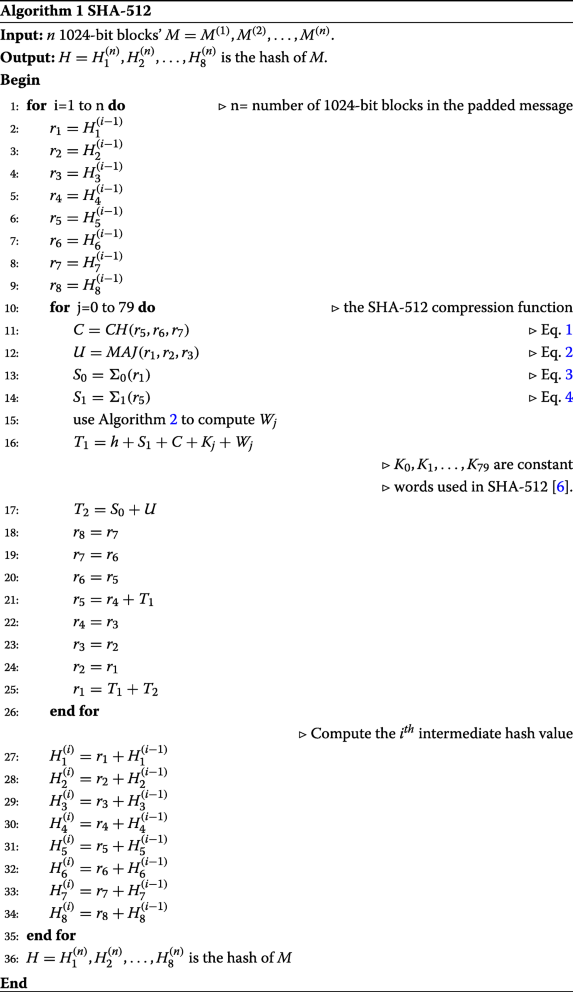The algorithm of SHA-512 is given in Algorithm 1. Now, we define the logical function used in Algorithm 1:

$$\begin{array}{*{20}l} CH(r_{1},r_{2},r_{3}) = (r_{1}\wedge r_{2}) \oplus (\neg r_{1}\wedge r_{3}) \end{array}$$
(1)
$$\begin{array}{*{20}l} MAJ(r_{1},r_{2},r_{3}) = (r_{1}\wedge r_{2}) \oplus (r_{1}\wedge r_{3}) \oplus (r_{2}\wedge r_{3}) \end{array}$$
(2)
$$\begin{array}{*{20}l} \Sigma_{0}(r_{1})= S^{28}(r_{1})\oplus S^{34}(r_{1}) \oplus S^{39}(r_{1}) \end{array}$$
(3)
$$\begin{array}{*{20}l} \Sigma_{0}(r_{1})= S^{14}(r_{1})\oplus S^{18}(r_{1}) \oplus S^{41}(r_{1}) \end{array}$$
(4)

The following algorithm, is to compute Wj.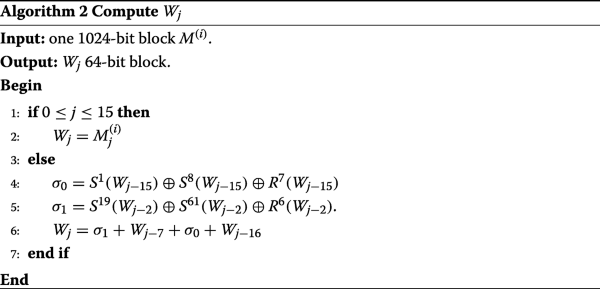## DNSHA-2

In this section, we propose modern operations on nucleotides that mimic the bitwise operations used in SHA-2 and can therefore be used to mimic all members of SHA-2, i.e., to give a new version of SHA-2 called DNSHA-2. This section contains seven subsections. In the “DNA coding” section, we give how to represent data in artificial DNA sequences. In the “Basic DNA-nucleotide operations” section, we present the nucleotide operations that mimic the bitwise operations (NOT, AND, OR, XOR). In the “DNA right and left shift” and “DNA right rotation” sections, we show how to implement the nucleotide operations $$\bar {R}^{k}, \bar {L}^{k}$$, and $$\bar {S}^{k}$$ which mimic the bitwise operations (shown in Table 1), Rk,Lk, and Sk, respectively. The nucleotide operation that mimic the word-wise addition (mod 264) is given in the “DNA-nucleotide addition (mod 264)” section. In the “DNA initialization and preprocessing” section, we show how initialization and preprocessing operations, especially in SHA-512, are imitated in DNA computing. In the following, sometimes, we refer to any choice of the nucleotide bases (A, C, G, or T) by the symbols xi,yi, and zi (or $$x_{i}^{\prime }, y_{i}^{\prime }$$, or $$z_{i}^{\prime }$$).

### DNA coding

In classical computing, data is stored in the binary form (sequence of bytes). There are results  which encode the binary data in a DNA sequence, where the two binary digits “00,” “01,” “10,” and “11” are transformed into the nucleotides A, C, G, and T, respectively. For example, the binary string “01001110” is transformed into the nucleotides “CATG.”

We conclude this by defining the transformation λ:

$$\lambda(e_{i+1}e_{i})=\left\{\begin{array}{ll} A, & \text{if}\ e_{i+1}e_{i}=00; \\ C, & \text{if}\ e_{i+1}e_{i}=01; \\ G, & \text{if}\ e_{i+1}e_{i}=10; \\ T, & \text{if}\ e_{i+1}e_{i}=11. \end{array} \right.$$

Algorithm 3 describes the representation of a data in an artificial DNA sequence. Since the byte (8-bit) is the commonly used data storage unit, we suppose in Algorithm 3 (also, in this article) that the binary data is of an even number of bits.We give the following example to illustrate steps of Algorithm 3.

### Example 1

Let e=(100111)2 be a binary data. The DNA nucleotides of e gives the artificial DNA sequence α=GCT since:

1. 1.

At i=0,x0=λ(11)=T,

2. 2.

At i=1,x1=λ(01)=C,

3. 3.

At i=2,x2=λ(10)=G.

Algorithm 4 shows how to decode binary data from an artificial DNA sequence. Note that in the following algorithm we use λ−1 to give the inverse transformation of λ.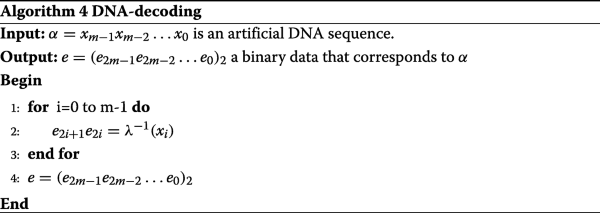We give the following example to illustrate steps of Algorithm 4.

### Example 2

Let α=GCT be an artificial DNA sequence. The binary data of α gives e=(100111)2 since:

1. 1.

At i=0,e1e0=λ−1(T)=11,

2. 2.

At i=1,e3e2=λ−1(C)=01,

3. 3.

At i=2,e5e4=λ−1(G)=10.

### Basic DNA-nucleotide operations

In literatures , the nucleotide operations that imitate bitwise operations (NOT, AND, OR, XOR) are defined. The symbols (¬,,,) are commonly used to express the bitwise operations (NOT, AND, OR, XOR), respectively. Throughout this paper, the symbols $$(\bar {\neg },\bar {\wedge },\bar {\vee },\bar {\oplus })$$ are used to give the nucleotide operations that imitate the bitwise operations (NOT, AND, OR, XOR), respectively. Note that we are putting a bar sign over most of the DNA operations or above the DNA terms to differ from bitwise operations.

The nucleotide operation $$\bar {\neg }$$ is defined as:

$$\begin{array}{*{20}l} \bar{\neg}A&= T \\ \bar{\neg}C&= G \end{array}$$

In literatures , the nucleotide operations between two nucleotides x and y are defined as in Table 3

### DNA right and left shift

In this subsection, we propose two new operations on DNA sequence that used to mimic the right and left shift by k bits. Let α=xm−1xm−2x0 be a DNA sequence and e=(e2m−1e2m−2e0)2 be the binary data encoded in α. We have to mimic the operation Rk(e) (right shift by k<2m bits) in SHA-2 to be $$\bar {R}^{k}(\alpha)$$ in DNSHA-2. In this regard, we take into consideration whether k is an even number or odd. In case of k is an even number, the operation Rk(e) can be imitated in α by deleting k/2 nucleotides from right and then appending k/2 nucleotides A from left. Therefore,

$$\begin{array}{*{20}l} \bar{R}^{k}(\alpha)= \underbrace{A A \ldots A}_{\frac{k}{2} nucleotides} x_{m-1} \ldots x_{k/2} \end{array}$$

For example, if α=TAGC, e=(11001001)2, and k=4, then

$$\begin{array}{*{20}l} R^{4}(e) &=00001100 \end{array}$$
(5)
$$\begin{array}{*{20}l} \bar{R}^{4}(\alpha)&= AATA \end{array}$$
(6)

In case of k is an odd number, the operation $$\bar {R}^{k}(\alpha)$$ can be computed in two steps. The first step is calculating $$\bar {R}^{k-1}(\alpha)$$ since k−1 is even. The second step is calculating the right shift by one bit in DNA sequence where we denote to this operation as RSOB(α) and define it in Algorithm 5.

Let α=xm−1xm−2x0 be an artificial DNA sequence and λ−1(xi)=e2i+1e2i. Then, RSOB(α) is ym−1ym−2y0, where λ−1(yi)=e2i+2e2i+1 for i=0,1,…,m−2 and λ−1(ym−1)=0e2m−1. To illustrate how to perform this step, we give the following notes:

1. 1.

If β is a DNA sequence of m nucleotides G, then $$\alpha \bar {\wedge } \beta$$ yields nucleotides zm−1zm−2z0, where λ−1(zi)=e2i+10 for i=0,1,…m−1, i.e., zi is either nucleotide A or G.

2. 2.

If α=Axm−1xm−2x1 and β is a DNA sequence of m nucleotides C, then $$\alpha ^{\prime } \bar {\wedge } \beta ^{\prime }$$ yields nucleotides $$A z^{\prime }_{m-1} \ldots z^{\prime }_{1},$$ where $$\lambda ^{-1}\left (z^{\prime }_{i}\right) = 0 e_{2i}$$ for i=1,2,…m−1, i.e., $$z^{\prime }_{i}$$ is either nucleotide A or C.

3. 3.

Therefore, we need to define the new nucleotide operation $$\bar {\boxtimes }$$ as follows:

If λ−1(zi)=e2i+10 and λ−1(zi+1′)=0e2i+2, then $$\lambda ^{-1}\left (z_{i} \bar {\boxtimes } z'_{i+1}\right) = e_{2i+2} e_{2i+1}.$$ We define this nucleotide operation in Table 4.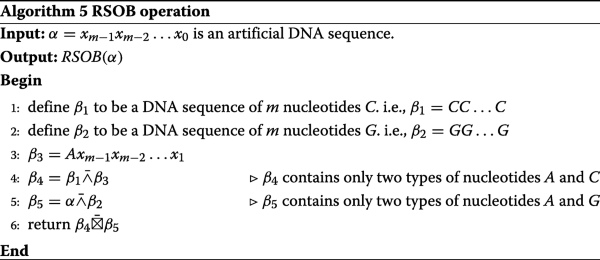The following example illustrates steps of Algorithm 5.

### Example 3

We use the same symbols in the algorithm. Let α=TAC be an artificial DNA sequence encoding the binary data e=(110001)2. We have β1=CCC,β2=GGG, and β3=ATA. Then, $$\beta _{4}=\beta _{1} \bar {\wedge } \beta _{3} =ACA$$ and $$\beta _{5}= \alpha \bar {\wedge } \beta _{2} = GAA.$$ The result is given by $$\beta _{4} \bar {\boxplus } \beta _{5} = CGA$$ encoding the binary data (011000)2.

We give the operation $$\bar {R}^{k}(\alpha)$$ in Algorithm 6.Similarly, we have to mimic the operation Lk(e) (left shift by k<2m bits) in SHA-2 to be $$\bar {L}^{k}(\alpha)$$ in DNSHA-2. In case of k is even, the operation Lk(e) can be imitated in α by deleting k/2 nucleotides from left and then appending k/2 nucleotides A from right. Therefore,

$$\begin{array}{*{20}l} \bar{L}^{k}(\alpha)= x_{k/2-1}\ldots x_{0} \underbrace{A A \ldots A}_{\frac{k}{2}nucleptides} \end{array}$$

For example, if α=TAGC, e=(11001001)2, and k=4, then

$$\begin{array}{*{20}l} L^{4}(e) &=10010000 \end{array}$$
(7)
$$\begin{array}{*{20}l} \bar{L}^{4}(\alpha)&= GCAA \end{array}$$
(8)

In case of k is odd, $$\bar {L}^{k}(\alpha)$$ can be computed in two steps. The first step is calculating $$\bar {L}^{k-1}(\alpha)$$ since k−1 is even. The second step is calculating the left shift by one bit in DNA sequence where we denote this operation as LSOB(α) and define it in Algorithm 7.

Let α=xm−1xm−2x0 be an artificial DNA sequence and λ−1(xi)=e2i+1e2i. Then, LSOB(α) is ym−1ym−2y0, where λ−1(yi)=e2ie2i−1 for i=1,2,…,m−1 and λ−1(y0)=e00.The following example illustrates steps of Algorithm 7.

### Example 4

We use the same symbols in the algorithm. Let α=GTC be an artificial DNA sequence encoding the binary data e=(101101)2. We have β1=CCC,β2=GGG, and β3=TCA. Then, $$\beta _{4}=\beta _{2} \bar {\wedge } \beta _{3} =GAA$$ and $$\beta _{5}= \alpha \bar {\wedge } \beta _{1} = ACC.$$ The result is given by $$\beta _{4} \bar {\boxplus } \beta _{5} = CGG$$ encoding the binary data (011010)2.

We give the operation $$\bar {L}^{k}(\alpha)$$ in Algorithm 8.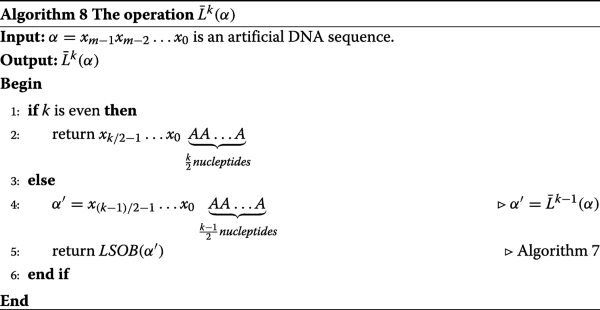### DNA right rotation

In this subsection, we introduce a new operation on DNA sequence that used to mimic the right rotation by k bits. In Algorithm 9, we give the operation $$\bar {S}^{k}(\alpha)$$ on DNA sequence α to imitate the operation Sk(e) (right rotation by k bits), where e is the binary data encoded in α.

Let α=xm−1xm−2x0 be a DNA sequence and e=(e2m−1e2m−2e0)2 be the binary data encoded in α. To compute $$\bar {S}^{k}(\alpha),$$ we first compute $$\bar {R}^{k}(\alpha)$$ using Algorithm 6 and then compute $$\bar {L}^{2m-k}(\alpha)$$ using Algorithm 8. Therefore, $$\bar {S}^{k}(\alpha)= \bar {R}^{k}(\alpha) \bar {\vee } \bar {L}^{2m-k}(\alpha).$$The following example illustrates steps of Algorithm 9.

### Example 5

We use the same symbols in the algorithm. Let α=AGT be an artificial DNA sequence encoding the binary data e=(001011)2 and k=4. We have $$\beta _{1}=\bar {R}^{4}(\alpha)= AAA,$$ and $$\beta _{2}=\bar {L}^{2}(\alpha)=GTA.$$ The result is given by $$\beta _{1} \bar {\vee } \beta _{2} = GTA$$ encoding the binary data (101100)2.

### DNA-nucleotide addition (mod 264)

In this subsection, we mimic word-wise addition (mod 264). We use the symbol $$\boxplus$$ to express nucleotide addition. In Table 5, the addition of two nucleotides x and y takes the form:

$$(z,\epsilon)= x \boxplus y$$

where z is the addition of two nucleotides x and y, and ε is called the carry nucleotide.

In Algorithm 10, we mimic the binary addition (mod 264). Note that the binary sequence of 64 bits can be encoded in a DNA sequence of 32 nucleotides. Therefore, in Algorithm 10, we have the inputs which are two DNA sequences each of 32 nucleotides.

We use the symbol $$\boxplus$$ between two DNA sequences each of 32 nucleotides to express the nucleotide addition (mod 264) given in Algorithm 10.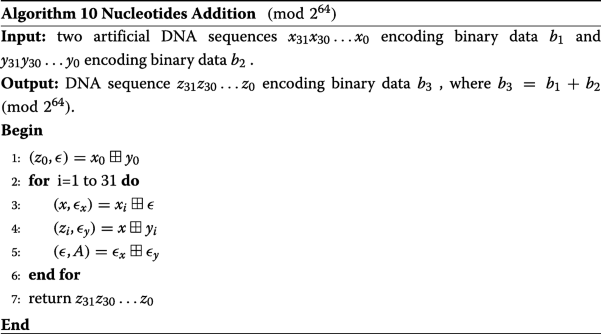Let

$$\begin{array}{*{20}l} \alpha_{1} &= TCTT TTCA GTAC AATT TGCA TAAC GTGT GGAA,\\ \alpha_{2} &= TGAT AGCT ATTC GATT TACT AAGC ATAT GTGA \end{array}$$

be inputs for Algorithm 10. The following example illustrates how to compute $$\alpha _{1} \boxplus \alpha _{2}.,$$ i.e., steps of Algorithm 10.

### Example 6

We use the same symbols in the algorithm. We have x0=A, y0=A, z0=A, and ε=A. Also, we have the following:

1. 1.

At i=1, x1=A, x=A, εx=A, y1=G, z1=G, εy=A, ε=A.

2. 2.

At i=2, x2=G, x=G, εx=A, y2=T, z2=C, εy=C, ε=C.

3. 3.

At i=3, x3=G, x=T, εx=A, y3=G, z3=C, εy=C, ε=C.

4. 4.

At i=4, x4=T, x=A, εx=C, y4=T, z4=T, εy=A, ε=C.

5. 5.

At i=5, x5=G, x=T, εx=A, y5=A, z5=T, εy=A, ε=A.

6. 6.

At i=6, x6=T, x=T, εx=A, y5=T, z5=G, εy=C, ε=C.

7. 7.

At i=7, x7=G, x=T, εx=A, y7=A, z7=T, εy=A, ε=A.

8. 8.

At i=8, x8=C, x=C, εx=A, y8=C, z8=G, εy=A, ε=A.

9. 9.

At i=9, x9=A, x=A, εx=A, y9=G, z9=G, εy=A, ε=A.

10. 10.

At i=10, x10=A, x=A, εx=A, y10=A, z10=A, εy=A, ε=A.

11. 11.

At i=11, x11=T, x=T, εx=A, y11=A, z11=T, εy=A, ε=A.

12. 12.

At i=12, x12=A, x=A, εx=A, y12=T, z12=T, εy=A, ε=A.

13. 13.

At i=13, x13=C, x=C, εx=A, y13=C, z13=G, εy=A, ε=A.

14. 14.

At i=14, x14=G, x=G, εx=A, y14=A, z14=G, εy=A, ε=A.

15. 15.

At i=15, x15=T, x=T, εx=A, y15=T, z15=G, εy=C, ε=C.

16. 16.

At i=16, x16=T, x=A, εx=C, y16=T, z16=T, εy=A, ε=C.

17. 17.

At i=17, x17=T, x=A, εx=C, y17=T, z17=T, εy=A, ε=C.

18. 18.

At i=18, x18=A, x=C, εx=A, y18=A, z18=C, εy=A, ε=A.

19. 19.

At i=19, x19=A, x=A, εx=A, y19=G, z19=G, εy=A, ε=A.

20. 20.

At i=20, x20=C, x=C, εx=A, y20=C, z20=G, εy=A, ε=A.

21. 21.

At i=21, x21=A, x=A, εx=A, y21=T, z21=T, εy=A, ε=A.

22. 22.

At i=22, x22=T, x=T, εx=A, y22=T, z22=G, εy=C, ε=C.

23. 23.

At i=23, x23=G, x=T, εx=A, y23=A, z23=T, εy=A, ε=A.

24. 24.

At i=24, x24=A, x=A, εx=A, y24=T, z24=T, εy=A, ε=A.

25. 25.

At i=25, x25=C, x=C, εx=A, y25=C, z25=G, εy=A, ε=A.

26. 26.

At i=26, x26=T, x=T, εx=A, y26=G, z26=C, εy=C, ε=C.

27. 27.

At i=27, x27=T, x=A, εx=C, y27=A, z27=A, εy=A, ε=C.

28. 28.

At i=28, x28=T, x=A, εx=C, y28=T, z28=T, εy=A, ε=C.

29. 29.

At i=29, x29=T, x=A, εx=C, y29=A, z29=A, εy=A, ε=C.

30. 30.

At i=30, x30=C, x=G, εx=A, y30=G, z30=A, εy=C, ε=C.

31. 31.

At i=31, x31=T, x=A, εx=C, y31=T, z30=T, εy=A, ε=C.

Thus, the result is the DNA sequence:

$$TAAT ACGT TGTG GCTT GGGT TAGG TGTT CCGA.$$

### DNA initialization and preprocessing

Since the initialization and preprocessing operations in the hash functions belonging to SHA-2 are almost similar, but differ only in initial values, we will focus on these operations for SHA-512 to be imitated in DNA computing. We give DNSHA-512 as the member of DNSHA-2 that mimics SHA-512 formed on an artificial DNA sequence.

The initial hash value H(0) is encoded in the DNA sequence $$\bar {H}^{(0)}$$ as in Table 6.

In this paper, we suppose that a binary data encoded in a DNA sequence is of an even number of bits. This is because, in the usual way, binary data are stored in some number of bytes (8-bit unit). In the following, we need to mimic the beginning computation in SHA-512 to be done similarly in DNSHA-512:

1. 1.

Pad the DNA sequence (supposed to be hashed) as follows: Suppose the length of the DNA sequence is m nucleotides. We append the nucleotide G to the end of the sequence, and after that k nucleotides of type A, where k is the minimal solution (non-negative) to the relation m+2+k≡448 (mod 512). Next, to this append, we add a DNA sequence of 64 nucleotides encoded the binary data of the value of 2m. We have the length of the padded DNA sequence which is a multiple of 512 nucleotides.

2. 2.

We parse the DNA sequence into n 512-nucleotide blocks’ $$\bar {M}^{(1)}, \bar {M}^{(2)},$$ …,$$\bar {M}^{(n)}.$$ The first 32 nucleotides of nucleotide block i are denoted $$\bar {M}_{0}^{(i)}$$, the next 32 nucleotides are $$\bar {M}_{1}^{(i)}$$, and so on up to $$\bar {M}_{15}^{(i)}$$. The nucleotide block i$$\bar {M}^{(i)}$$ (of 512 nucleotides) in DNSHA-512 has to imitate the 1024-bit block M(i) in SHA-512. Therefore, the 32 nucleotides of $$\bar {M}_{j}^{(i)}$$ have to be the DNA sequence that encodes $$M_{j}^{(i)}.$$

To show how to prepare the DNA sequence to be hashed, we give Example 7.

### Example 7

The binary data of the message “BOB” are “01000010 01001111 01000010.” This binary data is encoded in the DNA sequence “CAAGCATTCAAG” with m=12. By appending the nucleotide G to the end of this sequence, we get “CAAGCATTCAAG G.” Solving the equation 12+2+k≡448 (mod 512), we have k=434. Therefore, preparing the DNA sequence, we get:

$$\begin{array}{*{20}l}{} CAAGCATTCAAG\ G\ \underbrace{A A \ldots A}_{\text{434 nucleotides}}\ \underbrace{AAAAAAAAAAAAAAAAAAAAAAAAAAAAACGA}_{\text{64 nucleotides encode the binary of 24} } \end{array}$$

The 32 nucleotides of $$\bar {M}_{j}^{(1)}, j=0,1,\ldots, 15$$ are given as: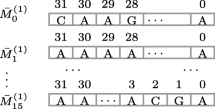### DNSHA-512

We give Algorithm 11 for DNSHA-512 that mimics Algorithm 1.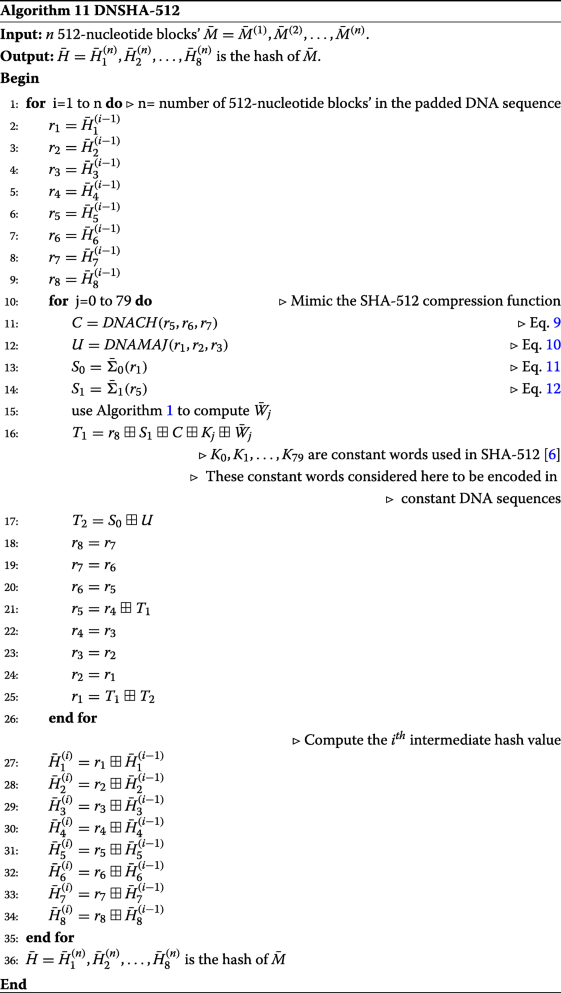Now, we define functions used in Algorithm 11 (DNA functions):

$$\begin{array}{*{20}l} DNACH(r_{1},r_{2},r_{3})= (r_{1}\bar{\wedge}r_{2}) \bar{\oplus} (\bar{\neg} r_{1} \bar{\wedge} r_{3}) \end{array}$$
(9)
$$\begin{array}{*{20}l} DNAMAJ(r_{1},r_{2},r_{3})=(r_{1}\bar{\wedge}r_{2}) \bar{\oplus} (r_{1} \bar{\wedge} r_{3}) \bar{\oplus} (r_{2} \bar{\wedge} r_{3}) \end{array}$$
(10)
$$\begin{array}{*{20}l} \bar{\Sigma}_{0}(\alpha) = \bar{S}^{28}(\alpha) \bar{\oplus} \bar{S}^{34}(\alpha) \bar{\oplus} \bar{S}^{39}(\alpha) \end{array}$$
(11)
$$\begin{array}{*{20}l} \bar{\Sigma}_{1}(\alpha) = \bar{S}^{14}(\alpha) \bar{\oplus} \bar{S}^{18}(\alpha) \bar{\oplus} \bar{S}^{41}(\alpha) \end{array}$$
(12)

Now, we give the algorithm needed to compute $$\bar {W}_{j}.$$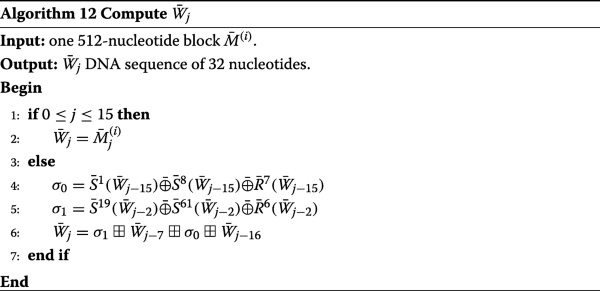## Implementation

This section, presents an implementation of DNSHA-512. Typically, all members of SHA-2 can similarly be implemented on an artificial DNA sequence. In Table 7, we consider some metrics to evaluate DNSHA-512 compared to SHA-512.

We made a computer program that simulates each step of DNSHA-512. Then, we apply the program to hash two types of data: text and image.

The text used for the hash is “BOB.” As previously stated in Example 7, the binary data for this message is encoded in the DNA sequence “CAAGCATTCAAG.” After padding the DNA sequence, we get:

$${}CAAGCATTCAAG \ G \ \underbrace{A A \ldots A}_{\text{434 nucleotides}} \ \underbrace{AAAAAAAAAAAAAAAAAAAAAAAAAAAAACGA}_{\text{64 nucleotides encode the binary of 24}}$$

The hash of this message using DNSHA-512 is given by the 32 nucleotides of $$\bar {H}_{1}^{(1)}, \bar {H}_{2}^{(1)}, \ldots, \bar {H}_{8}^{(1)}$$ as follows:

 31 30 29 28 27 26 25 24 23 22 21 20 19 18 17 16 15 14 13 12 11 10 9 8 7 6 5 4 3 2 1 0 $$\bar {H}_{1}^{(1)}$$ T T T C A G G A A T A C C A A A A C A C G C G A C G G T T C C A $$\bar {H}_{2}^{(1)}$$ G A T G G T T C G T C T C A A A C C C A G A C T G C C C C C A G $$\bar {H}_{3}^{(1)}$$ G C T A G T T C A T C T G G A C A G T C C G C T C T C T C A G G $$\bar {H}_{4}^{(1)}$$ G G T G G C C A C G G T C C C G A C C C A T A G A T G A C A G G $$\bar {H}_{5}^{(1)}$$ G C T A C A T T A C C G T C A T C T C G G T T C C G T T T C C A $$\bar {H}_{6}^{(1)}$$ A C G A C C A G A G T T G A A G G T A T C A T T T A C T C C T C $$\bar {H}_{7}^{(1)}$$ T T G A C G C T C C T A G A T G A C T T T G A C T G C A C G C T $$\bar {H}_{8}^{(1)}$$ A C T T T A G A A A A G T C T A T T G A A G A C T C G T A T G C

The corresponding hash of this message using SHA-512 is given by 64-bit words of $$H_{1}^{(1)}, H_{2}^{(1)}, \ldots, H_{8}^{(1)}$$ as follows:

 $$H_{1}^{(1)}$$ fd28314011986bd4 $$H_{2}^{(1)}$$ 8ebdb74054879552 $$H_{2}^{(1)}$$ 9cbd37a12d67774a $$H_{4}^{(1)}$$ ae946b561532384a $$H_{5}^{(1)}$$ 9c4f16d376bd6fd4 $$H_{6}^{(1)}$$ 18522f82b34fc75d $$H_{7}^{(1)}$$ f8675c8e1fe1e467 $$H_{8}^{(1)}$$ 1fc802dcf821db39

The image used for the hash is the lake image declared in Fig. 1.

This image has 4,200,848 bits. After padding, the binary data of this image has 4103 message blocks (1024-bit). The hash of this image using DNSHA-512 is given by the 32 nucleotides of $$\bar {H}_{1}^{(4103)}, \bar {H}_{2}^{(4103)}, \ldots, \bar {H}_{8}^{(4103)}$$ as follows:

 31 30 29 28 27 26 25 24 23 22 21 20 19 18 17 16 15 14 13 12 11 10 9 8 7 6 5 4 3 2 1 0 $$\bar {H}_{1}^{(4103)}$$ A A G G T G T C C G C C C T T C T G C G G C T T T C G A T G C G $$\bar {H}_{2}^{(4103)}$$ T C T A G T T C G T C A C C C C G G T T T A T G C C A C T G C T $$\bar {H}_{3}^{(4103)}$$ G G T C T A A C G C T T G C A C G G A G G G C G A A T C A C C T $$\bar {H}_{4}^{(4103)}$$ G G T T G A A T C G C T G C G C T C C G A T G T C A C C A G G A $$\bar {H}_{5}^{(4103)}$$ A C C A T C A A T T A T A G A T G T T C C A C T C C G A G G A G $$\bar {H}_{6}^{(4103)}$$ C A T A C A T T C A T G T C A T C A T C T G C G G C T C A T G C $$\bar {H}_{7}^{(4103)}$$ A A G G T T G C T T A G C T G A G A A C A A G T T G G G A C G C $$\bar {H}_{8}^{(4103)}$$ A T C T C G A A A A G T A G T T G C G G T T A A C G A T G A G T

The corresponding hash of this image using SHA-512 is given by 64-bit words of $$H_{1}^{(4103)}, H_{2}^{(4103)}, \ldots, H_{8}^{(4103)}$$ as follows:

 $$H_{1}^{(4103)}$$ 0aed657de69fd8e6 $$H_{2}^{(4103)}$$ dcbdb455afce51e7 $$H_{2}^{(4103)}$$ adc19f91a2a60d17 $$H_{4}^{(4103)}$$ af836799d63b4528 $$H_{5}^{(4103)}$$ 14d0f323bd4758a2 $$H_{6}^{(4103)}$$ 4c4f4ed34de69d39 $$H_{7}^{(4103)}$$ 0af9f278810bea19 $$H_{8}^{(4103)}$$ 37600b2f9af0638b

## Conclusion

We have presented the implementation of SHA-2 using DNA data processing. To the best of our knowledge, this result is the first attempt to model a standard hash function using DNA data processing. We have shown how to encode binary data into a DNA sequence, and we have given nucleotide operations that mimic the bitwise operations used in SHA-2. In particular, we have presented the DNA operations $$\bar {R}^{k}(\alpha), \bar {L}^{k}(\alpha),$$ and $$\bar {S}^{k}(\alpha)$$ that used to mimic the bitwise operations Rk(e),Lk(e), and Sk(e), where e (binary data) is encoded in the the DNA sequence α. Therefore, this work can be used to mimic any hash algorithm of its bitwise operations limited to bitwise operations specified in SHA-2. Similarly, the nucleotide operations proposed in this result can be exploited to lead to a preliminary result to perform SHA-3 on DNA sequences.

Not applicable.

## References

1. Aoki, K., Guo, J., Matusiewicz, K., Sasaki, Y., Wang, L.: Preimages for step-reduced SHA-2. In: Advances in Cryptology - ASIACRYPT 2009, 15th International Conference on the Theory and Application of Cryptology and Information Security, Tokyo, Japan, December 6-10, 2009. Proceedings, Vol. 5912 of Lecture Notes in Computer Science, pp. 578–597. Springer (2009). https://doi.org/10.1007/978-3-642-10366-7_34.

2. Indesteege, S., Mendel, F., Preneel, B., Rechberger, C.: Collisions and other non-random properties for step-reduced SHA-256. In: Selected Areas in Cryptography, pp. 276–293. Springer (2009). https://doi.org/10.1007/978-3-642-04159-4_18.

3. Kelsey, J., Kohno, T.: Herding hash functions and the nostradamus attack. In: Advances in Cryptology - EUROCRYPT 2006, pp. 183–200. Springer (2006). https://doi.org/10.1007/11761679_12.

4. Sanadhya, S., Sarkar, P.: New collision attacks against up to 24-step SHA-2. In: Progress in Cryptology-INDOCRYPT 2008, pp. 91–103. Springer (2008). https://doi.org/10.1007/978-3-540-89754-5_8.

5. Menezes, A. J., van Oorschot, P. C., Vanstone, S. A.: Handbook of Applied Cryptography, CRC Press, Inc., USA (1996).

6. N.I. of Standards, Technology, FIPS PUB 180-4: Secure Hash Standard, pub-NIST (2012). http://csrc.nist.gov/publications/fips/fips180-4/fips-180-4.pdf.

7. N.I. of Standards, Technology, SHA-3 Standard: Permutation-Based Hash and Extendable-Output Functions: FiPS PUB 202, pub-NIST (2015). https://books.google.com.eg/books?id=hCwatAEACAAJ.

8. Friedman, M., Rogers, Y., Boyce-Jacino, M.: Gene pen devices for array printing, WO Patent App, No. 6235473 (2000). http://www.freepatentsonline.com/6235473.html.

9. Kimoto, M., Matsunaga, K., Hirao, I. I.: DNA aptamer generation by genetic alphabet expansion SELEX (ExSELEX) using an unnatural base pair system. Springer, New York (2016).

10. Calladine, C., Drew, H., Luisi, B., Travers, A.: Understanding DNA: The Molecule and How itWorks. 3rd ed. Academic Press, Cambridge (2004).

11. Watson, J.: Molecular biology of the gene, Benjamin/Cummings (1987). https://books.google.com.eg/books?id=cM0fAQAAIAAJ.

12. Atito, A., Khalifa, A., Rida, S. Z., Khalifa, A.: DNA-based data encryption and hiding using playfair and insertion techniques. J. Commun. Comput. Eng. 2, 44–49 (2012).

13. Guo, C., Chang, C., Wang, Z.: A new data hiding scheme based on DNA sequence. Int. Innov. J. Comput. Inf. Control. 8, 1–11 (2012).

14. Khalifa, A.: Lsbase: a key encapsulation scheme to improve hybrid crypto-systems using DNA steganography. In: 2013 8th International Conference on Computer Engineering & Systems (ICCES), pp. 105–110 (2013). https://doi.org/10.1109/icces.2013.6707182.

15. Khalifa, A, Atito, A: High-capacity DNA-based steganography. In: 8th International Conference on Informatics and Systems. IEEE (2012). BIO–76–BIO–80.

16. Skariya, M., Varghese, M.: Enhanced double layer security using RSA over DNA based data encryption system. Int J Comput Sci Eng Technol. 4, 746–750 (2013).

17. Taur, J., Lin, H., Lee, H., Tao, C.: Data hiding in DNA sequences based on table lookup substitution. Int J Innov Comput Inf Control. 8, 6585–6598 (2012).

18. UbaidurRahmana, N. H., Balamuruganb, C., Mariappanab, R.: A novel DNA computing based encryption and decryption algorithm. Procedia Comput. Sci. 46, 463–475 (2015).

19. UbaidurRahmana, N. H., Balamuruganb, C., Mariappanab, R.: A novel string matrix data structure for DNA encoding algorithm. Procedia Comput. Sci. 46, 820–832 (2015).

20. Adleman, L.: Molecular computation of solutions to combinatorial problems. Science. 266(11), 1021–1024 (1994).

21. Bahig, H. M., Nassr, D. I.: DNA-based AES with silent mutations. Arab. J. Sci. Eng. 44, 1–15 (2018). https://doi.org/10.1007/s13369-018-3520-8.

22. Boneh, D., Dunworth, C., Lipton, R., Sgall, J: On the computational power of DNA. Discret. Appl. Math. 71(1-3), 79–94 (1996).

23. Kari, L., Seki, S., Sosík, P.: DNA Computing—Foundations and Implications. Springer, Berlin (2012).

24. Lipton, R.: Using DNA to solve np-complete problems. Science. 268, 542–545 (1995).

25. Boneh, D., Dunworth, C., Lipton, R.: Breaking DES using a molecular computer. In: DNA Based Computers, Proceedings of a DIMACS Workshop, Princeton, New Jersey, USA, April 4, 1995, pp. 37–66 (1995). https://doi.org/10.1090/dimacs/027/04.

26. Abbasy, M., Manaf, A., Shahidan, M.: Data Hiding Method Based on DNA Basic Characteristics. Springer (2011). https://doi.org/https://doi.org/10.1007/978-3-642-22603-8_5.

27. Abbasy, M., Nikfard, P., Ordi, A., Torkaman, M.DNA base data hiding algorithm. 1, 183–193 (2012).

28. Gehani, A., LaBean, T., Reif, J.: DNA-based Cryptography. Springer, Berlin (2004).

29. Hamed, G., Marey, M., El-Sayed, S. S., Tolba, F.: DNA Based Steganography: Survey and Analysis for Parameters Optimization. Springer, Cham (2016). https://doi.org/10.1007/978-3-319-21212-8_3.

30. Tang, Q., Ma, G., Zhang, W., Yu, N.: Reversible data hiding for DNA sequences and its applications. Int. Digit. J. Crime For. 6(4), 1–13 (2014).

31. Cui, G., Qin, L., Wang, Y., Zhang, X.: An encryption scheme using DNA technology. In: Third International Conference on Bio-Inspired Computing: Theories and Applications, pp. 37–42 (2008). https://doi.org/10.1109/bicta.2008.4656701.

32. Sabry, M., Hashem, M., Nazmy, T., Khalifa, M. E.: Design of DNA-based advanced encryption standard (AES). In: 2015 IEEE Seventh International Conference on Intelligent Computing and Information Systems (ICICIS), pp. 390–397 (2015). https://doi.org/10.1109/intelcis.2015.7397250.

33. Xin-she, L., Lei, Z., Yu-pu, H.: A novel generation key scheme based on DNA. In: International Conference on Computational Intelligence and Security, pp. 264–266 (2008). https://doi.org/10.1109/cis.2008.113.

34. Wang, X., Zhang, Q.: DNA computing-based cryptography. In: 2009 Fourth International on Conference on Bio-Inspired Computing, pp. 1–3 (2009). https://doi.org/10.1109/bicta.2009.5338153.

## Acknowledgements

We are grateful to Hatem M. Bahig for his support, valuable comments, and remarks. Furthermore, we are thankful to the referees for their precious comments, which lead to the improvement of the paper.

Not applicable

## Author information

Authors

### Contributions

DIN is the only author of this article, and he has performed all the analysis, verifications, and completions of the results included in this article. The author read and approved the final manuscript.

### Corresponding author

Correspondence to Dieaa I. Nassr.

## Ethics declarations

### Competing interests

The author declares that he has no competing interests.